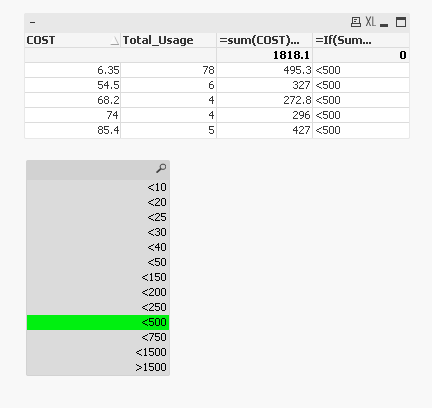# New to QlikView

Discussion board where members can get started with QlikView.

Announcements
QlikWorld, June 24-25, 2020. Free virtual event for DI and DA gurus. Register Now
cancel
Showing results for
Did you mean:
HighlightedContributor III

## Grouping Expression Data by a range

Hi All,

Wondering whether someone can give some help.

How can I group together data output from an expression into a range.

e.g

expression output below, I would like this to be grouped like so: '0','<1','<2','<3' etcThanks.

Tags (2)
20 Replies
HighlightedMVP

## Re: Grouping Expression Data by a range

Check here

Buckets

HighlightedContributor III

## Re: Grouping Expression Data by a range

Will this work even if i want to keep the original value also?

Example of an expression - =sum((TOTAL_USAGE)/12)/(-ONHAND_QTY)

I have around 6 of these 'buckets' which will need to be created, all with different expressions but some of the same variables.

HighlightedMVP

## Re: Grouping Expression Data by a range

You want to keep original values? Not sure I follow? Would you be able to share a sample?

HighlightedContributor III

## Re: Grouping Expression Data by a range

Hi Sunny,

So sorry for the late response. I have attached a sample file with a quite rubbish attempt to explain what I am looking for. Would you be able to have a look?

Thanks!

HighlightedMaster III

## Re: Grouping Expression Data by a range

May be like this?

HighlightedMVP

## Re: Grouping Expression Data by a range

Something like this?=If(Sum(COST)*(Total_Usage) < 0, '<0',

If(Sum(COST)*(Total_Usage) = 0, '0',

If(Sum(COST)*(Total_Usage) < 5, '<5',

If(Sum(COST)*(Total_Usage) < 10, '<10',

If(Sum(COST)*(Total_Usage) < 15, '<15',

If(Sum(COST)*(Total_Usage) < 20, '<20',

If(Sum(COST)*(Total_Usage) < 25, '<25',

If(Sum(COST)*(Total_Usage) < 30, '<30',

If(Sum(COST)*(Total_Usage) < 40, '<40',

If(Sum(COST)*(Total_Usage) < 50, '<50',

If(Sum(COST)*(Total_Usage) < 100, '<100',

If(Sum(COST)*(Total_Usage) < 150, '<150',

If(Sum(COST)*(Total_Usage) < 200, '<200',

If(Sum(COST)*(Total_Usage) < 250, '<250',

If(Sum(COST)*(Total_Usage) < 500, '<500',

If(Sum(COST)*(Total_Usage) < 750, '<750',

If(Sum(COST)*(Total_Usage) < 1000, '<1000',

If(Sum(COST)*(Total_Usage) < 1500, '<1500', '>1500'))))))))))))))))))

HighlightedContributor III

## Re: Grouping Expression Data by a range

This is what I am looking for, however instead of it as another expression in my table, I would like it as a selection box such as this in excel.Even as a selection in say a list box would work.

HighlightedMVP

## Re: Grouping Expression Data by a range

Something like this?HighlightedContributor III

## Re: Grouping Expression Data by a range

That is exactly what I am looking for. I have done the below for my expression, but it is returning null. Can you see what is wrong?

=Aggr(

If((sum({1}TOTAL_USAGE)/12/only({1}-ONHAND_QTY)) < 0, Dual('<0', 1),

If((sum({1}TOTAL_USAGE)/12/only({1}-ONHAND_QTY)) = 0, Dual('0', 2),

If((sum({1}TOTAL_USAGE)/12/only({1}-ONHAND_QTY)) < 5, Dual('<5', 3),

If((sum({1}TOTAL_USAGE)/12/only({1}-ONHAND_QTY)) < 10, Dual('<10', 4),

If((sum({1}TOTAL_USAGE)/12/only({1}-ONHAND_QTY)) < 15, Dual('<15', 5),

If((sum({1}TOTAL_USAGE)/12/only({1}-ONHAND_QTY)) < 20, Dual('<20', 6),

If((sum({1}TOTAL_USAGE)/12/only({1}-ONHAND_QTY)) < 25, Dual('<25', 7),

If((sum({1}TOTAL_USAGE)/12/only({1}-ONHAND_QTY)) < 30, Dual('<30', 8),

If((sum({1}TOTAL_USAGE)/12/only({1}-ONHAND_QTY)) < 40, Dual('<40', 9),

If((sum({1}TOTAL_USAGE)/12/only({1}-ONHAND_QTY)) < 45, Dual('<45', 10),

If((sum({1}TOTAL_USAGE)/12/only({1}-ONHAND_QTY)) < 50, Dual('<50', 11),

If((sum({1}TOTAL_USAGE)/12/only({1}-ONHAND_QTY)) < 55, Dual('<55', 12),

If((sum({1}TOTAL_USAGE)/12/only({1}-ONHAND_QTY)) < 60, Dual('<60', 13),

If((sum({1}TOTAL_USAGE)/12/only({1}-ONHAND_QTY)) < 65, Dual('<65', 14),

If((sum({1}TOTAL_USAGE)/12/only({1}-ONHAND_QTY)) < 70, Dual('<70', 15), Dual('>70', 16)))))))))))))))), %Key1)